# High School Physics : Understanding Circular Motion

## Example Questions

### Example Question #1 : Circular Motion

Ellen is swinging a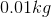yo-yo in a circular path perpendicular to the ground. The yo-yo moves in a clockwise direction with a constant speed of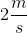.

What is the velocity of the yo-yo at the bottom of the circle?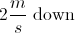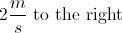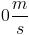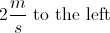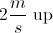Explanation:

Remember, when working with circular motion, the velocity is ALWAYS tangential to the circle. This means that, even though the speed is constant, the direction is always tangent to the edge of the circle. If the circle below represents the path of the yo-yo, and it moves in a clockwise direction, then the velocity at the bottom of the path will be to the left.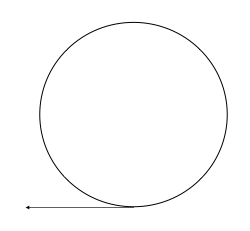The magnitude of the velocity is constant, so the final answer will be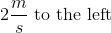.

### Example Question #2 : Circular Motion

Two children sit on a merry-go-round. One sits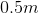from the center, and the other sits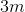from the center. If the children are in a straight line form the center, which child has a greater speed?

The child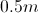from the center

We must know the acceleration to draw a conclusion

The child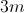from the center

We must know the period to draw a conclusion

They have the same speed

The childfrom the center

Explanation:

Velocity is given by distance per unit time.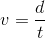When moving in a circle, the distance is the circumference, and each rotation takes exactly one period. We can substitute into the velocity formula.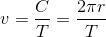If the children are in a straight line, that means that their periods (how long it takes to make one revolution) will be the same. The only thing that changes is, the distance from the center. Since radius is in the numerator, we can conclude that increasing the distance from the center will increase the velocity.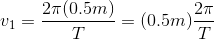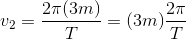### Example Question #1 : Understanding Circular Motion

Which of the following will increase the torque on a wrench, without changing the force applied?

Change the angle of rotation

Use a longer wrench

Use a shorter wrench

Use a wrench of a stronger material

None of these will change the torque generated

Use a longer wrench

Explanation:

The formula for torque is:If the force applied remains the same, then that means that the torque and the lever arm are directly proportional: when one increases the other increases as well. The length of the lever arm, in this problem, is the length of the wrench; thus, increasing the length of the wrench will increase the amount of torque generated, without requiring a change in the force applied.

### Example Question #1 : Circular Motion

A car driving on the highway is moving at 60 miles per hour. As the car nears an exit ramp, the car slows to 35 miles per hour, a speed that is maintained throughout the circular path of the exit ramp. What force is keeping the car on its path (i.e. in circular motion)?

Normal force

Momentum

Weight of the car (gravity)

Centripetal force

Centripetal force

Explanation:

The correct answer is centripetal force. In a free body diagram, this is the force that would be directed towards the center of the circular path; in circular motion, acceleration and net force are always in this direction.

Normal force and weight act perpendicular to the line that is directed towards the center of the circle, and will not prevent the car from traveling off the circular path. Momentum is not a measure of force and is not particularly relevant to this question.

### Example Question #1 : Circular Motion

A ball attached to a string is moving counterclockwise in a vertical circle. If the string is cut exactly at the point where the ball is at the top of its motion (the top of the circle), what direction will the ball move in initially?

Right

Downward

Left

Upward